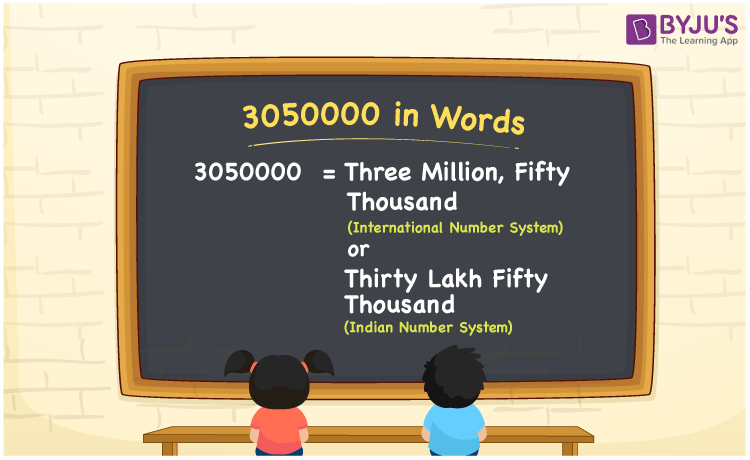# 3050000 in Words

According to the Indian Number System, the number 3050000 in words is thirty lakh fifty thousand. Whereas, the number name of 3050000 according to the International Number System is three million and fifty thousand. In this article, we are going to discuss the procedure of writing the number 3050000 using the place value system in detail.

 3050000 in Words (Indian Number System): Thirty Lakh Fifty Thousand  3050000 in Words (International Number System): Three Million and Fifty Thousand. Thirty Lakh Fifty Thousand in Numerical Form: 3050000.

## 3050000 in English Words## How to Write 3050000 in Words?

Go through the below table to learn the place values of 3050000.

 Ten-lakhs Lakhs Ten-thousands Thousands Hundreds Tens Ones 3 0 5 0 0 0 0

The expanded form of 3050000 is as follows:

= 3 × Ten lakh + 0 × Lakh + 5 × Ten thousand + 0 × Thousand + 0 × Hundred + 0 × Ten + 0 × One

= 3 × 1000000 + 0 × 100000 + 5 × 10000 + 0 × 1000 + 0 × 100 + 0 × 10 + 0 × 1

= 3000000 + 50000

= 3050000

= Thirty Lakh Fifty Thousand

Hence, 3050000 in words is thirty lakh fifty thousand (or) three million and fifty thousand.

3050000 in words – Thirty Lakh Fifty Thousand

Is 3050000 an odd number? – No

Is 3050000 an even number? – Yes

Is 3050000 a perfect square number? – No

Is 3050000 a perfect cube number? – No

Is 3050000 a prime number? – No

Is 3050000 a composite number? – Yes

## Frequently Asked Questions on 3050000 in Words

Q1

### How to write 3050000 in words according to the Indian Number System?

According to the Indian Number System, 3050000 in words is thirty lakh fifty thousand.

Q2

### Simplify 3000000 + 50000, and express it in words.

Simplifying 3000000 + 50000, we get 3050000. Hence, 3050000 in words is thirty lakh fifty thousand.

Q3

### How to write 3050000 in words according to the International Number System?

According to the International Number System, 3050000 in words is three million and fifty thousand.